### Captures d’écran iPhone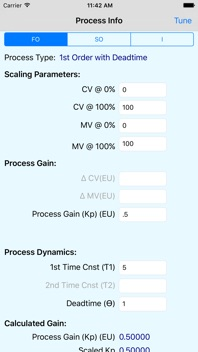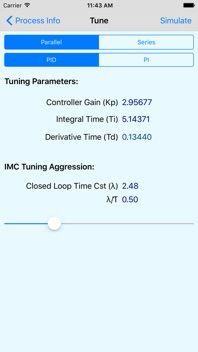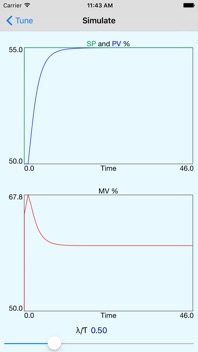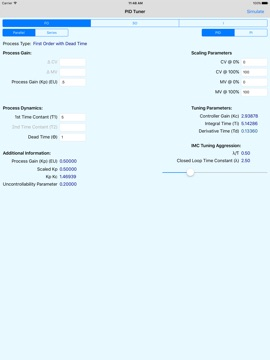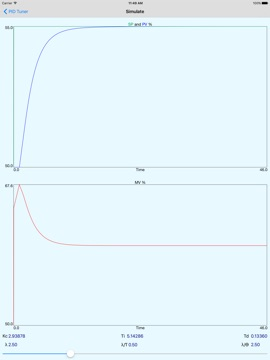### Description

PID Tuner uses the IMC (Internal Model Control) method to calculate PID tuning parameters. I’ve tried a lot of tuning methods over the years and have found this one to be the best. The app is written for the iPad to show all the information on one page.
The program calculates:
PID Parameters:
Controller Gain (Kc)
Integral Time (Ti)
Derivative Time (Td)
For process types
First Order with Dead Time (FO)
Second Order with Dead Time (SO)
Integrating or Long Time Constant with Dead Time (I)
For controller types
Parallel (non-interacting)
c(s) = Kc (1 + 1/(Ti s) + Td s)
Series (interacting)
c(s) = Kc (Ti s + 1)/(Ti s)(Td s + 1)
For controller modes
PID
PI
You can either enter the process gain (Kp) directly or enter the changes in the controlled virable (CV) and manipulated variable (MV).
Tuning aggression is adjusted using the slider to set the closed loop time constant to the process time constant ratio (λ/Ƭ).
The closed loop time constant (λ) is the time the controller is expected to reach set point (plus the dead time (ϴ)). Setting the closed loop time constant to process time constant ratio (λ/Ƭ) to 1.0 will make the MV change to its final value and allow the process to settle out to the setpoint. Setting (λ/Ƭ) greater than 1.0 will give a slower responce. Setting (λ/Ƭ) less than 1.0 will cause the MV to make a larger change and then reduce to its final value. Tight tuning would be to set the close loop time constant to the dead time.
Use the Integrating Process type (Ƭ = ∞) for both integrating processes or processes with long time constants. These processes use the maximum slope as the process gain. Tuning aggression for these processes is determined by setting the closed loop time constant to the dead time ratio (λ/ϴ). Once again, setting the closed loop time constat to the dead time gives tight control.
References:
“Probably the test simple PID tuning rules in the World,” Sigurd Skogestad, Jounal of Process Control, July 3, 2001
“Consider the generalized IMC-PID method for PID controller tuning of time-delay processes,” Y. Lee, S. Park, M. Lee, Hydrocarbon Processing, January 2006

Minor tweeks.

Richard H Street

### Informations

2.7

1,2 MB

Non

Oui

#### Classement

Classification : 4+

#### Compatibilité

iOS 13.6 ou plus récent

anglais

Pas de note

Pas d'entrée